2021 NBA Sixth Man of the Year Odds
-140
0.71 to 1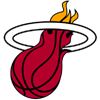Tyler Herro
47.6% implied probability

+1400
14 to 1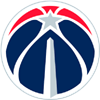Montrezl Harrell
5.4% implied probability

+2200
22 to 1Jordan Clarkson
3.5% implied probability

+2500
25 to 1Jalen Brunson
3.1% implied probability

+2500
25 to 1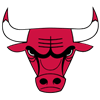Alex Caruso
3.1% implied probability

+2500
25 to 1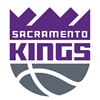Buddy Hield
3.1% implied probability

+3000
30 to 1Kelly Oubre
2.6% implied probability

+3000
30 to 1Dennis Schroder
2.6% implied probability

+3000
30 to 1Bobby Portis
2.6% implied probability

+3500
35 to 1Ricky Rubio
2.3% implied probability

+4000
40 to 1Jordan Poole
2.0% implied probability

+5000
50 to 1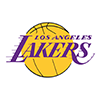Carmelo Anthony
1.6% implied probability

+5000
50 to 1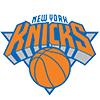Derrick Rose
1.6% implied probability

+6000
60 to 1T.J. McConnell
1.3% implied probability

+6000
60 to 1Patty Mills
1.3% implied probability

+10000
100 to 1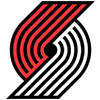Anfernee Simons
0.8% implied probability

+10000
100 to 1Pat Connaughton
0.8% implied probability

+10000
100 to 1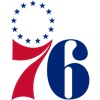Shake Milton
0.8% implied probability

+10000
100 to 1Talen Horton-Tucker
0.8% implied probability

+10000
100 to 1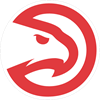Cam Reddish
0.8% implied probability

+15000
150 to 1Immanuel Quickley
0.5% implied probability

+15000
150 to 1Terance Mann
0.5% implied probability

+15000
150 to 1Cameron Johnson
0.5% implied probability

+15000
150 to 1Kelly Olynyk
0.5% implied probability

+15000
150 to 1Andre Drummond
0.5% implied probability

+15000
150 to 1Cameron Payne
0.5% implied probability

+15000
150 to 1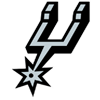Devin Vassell
0.5% implied probability

+15000
150 to 1Joe Ingles
0.5% implied probability

+20000
200 to 1Luke Kennard
0.4% implied probability

+20000
200 to 1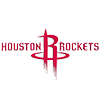Eric Gordon
0.4% implied probability

+20000
200 to 1Malik Monk
0.4% implied probability

+20000
200 to 1Malik Beasley
0.4% implied probability

+20000
200 to 1Furkan Korkmaz
0.4% implied probability

+20000
200 to 1Lonnie Walker
0.4% implied probability

+20000
200 to 1Matisse Thybulle
0.4% implied probability

+25000
250 to 1Dwight Howard
0.3% implied probability

+25000
250 to 1Kevin Huerter
0.3% implied probability

+25000
250 to 1Terrence Ross
0.3% implied probability

+30000
300 to 1Danilo Gallinari
0.3% implied probability

+30000
300 to 1Coby White
0.3% implied probability

+30000
300 to 1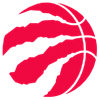Chris Boucher
0.3% implied probability

+50000
500 to 1Brandon Clarke
0.2% implied probability

+50000
500 to 1Marvin Bagley
0.2% implied probability

+50000
500 to 1Justise Winslow
0.2% implied probability

+50000
500 to 1Thaddeus Young
0.2% implied probability

+50000
500 to 1Davis Bertans
0.2% implied probability

+50000
500 to 1Facundo Campazzo
0.2% implied probability

+50000
500 to 1Aaron Holiday
0.2% implied probability

+50000
500 to 1Hamidou Diallo
0.2% implied probability

+50000
500 to 1Jaxson Hayes
0.2% implied probability

+50000
500 to 1Jarrett Culver
0.2% implied probability

+50000
500 to 1Otto Porter
0.2% implied probability

+50000
500 to 1Kenyon Martin
0.2% implied probability

+50000
500 to 1Juan Toscano-Anderson
0.2% implied probability

+50000
500 to 1Serge Ibaka
0.2% implied probability

+50000
500 to 1Deni Avdija
0.2% implied probability

+50000
500 to 1Tomas Satoransky
0.2% implied probability

+50000
500 to 1Terence Davis
0.2% implied probability

+50000
500 to 1Bruce Brown
0.2% implied probability